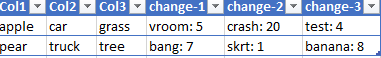cancel
Showing results for
Did you mean:Frequent Visitor

## Custom Function Looping Through Columns in Table

Hi All,

Been struggling for hours to figure out why my function is not working in the Power Query Editor. A quick synopsis of what I am trying to to:

• Find all columns where the header contains a specific character in a table
• In these specific columns, erase all text before a specific delimeter in each row
• Return the edited table

This is an example of what I would like my function to do to the data:

Table prior to functionTable after functionBelow is the code that I have created for a function to try to conduct these simple operations:

``````(Loop as number, Tbl as table) =>
let
ColMax = Table.ColumnCount(Tbl),
newTbl = if (Loop <= ColMax) and (Text.Contains(Table.ColumnNames(Tbl){Loop},"-")) then Table.TransformColumns(Tbl, {Table.ColumnNames(Tbl){Loop}, each Text.AfterDelimiter(_,": "), type text}) else Tbl,
CurrentCol = Loop + 1,
output =
if CurrentCol <= ColMax then @Function1(CurrentCol,newTbl)
else newTbl

in
output``````

Currently in the Advanced Editor screen it shows there are no syntax errors in my code, however, when I run it I get the following error:I need my code to be able to edit a dynamic number of columns, since the number of columns will always be changing for this specific table. The names of the columns will also change, but the ones I need to edit will always have the specific character "-" in it. If anyone has any guidance on how to solve this error it would be greatly appreciated!

P.S. this is my first ever post on here, so if I did not include enough detail or uploaded something incorrectly please let me know and I will modify this post ASAP. I am new to coding in Power Query but have a background in Python, and have spent hours trying to figure out the functions and syntax to use in here with little success.

1 ACCEPTED SOLUTIONSuper User

There's no reason to use loops here and your error is related to the Loop index. M is a functional language and works best when you define transformations rather than procedural code.

For simplicity, it's often easier to define a query instead of a function that needs to be called when first building something and then turn it into a function once you have it working. For example:

``````let
Source = Table.FromRows(Json.Document(Binary.Decompress(Binary.FromText("i45WSiwoyElV0lFKTiwCkulFicXFQLqsKD8/10rBFCQBFMqwUjAyALJLUotLrBRMlGJ1opUKUsE6SopKk7PBdCrImKTEvHQrBXMgqzi7CKjWECIGhFYKFkqxsQA=", BinaryEncoding.Base64), Compression.Deflate)), let _t = ((type nullable text) meta [Serialized.Text = true]) in type table [Col1 = _t, Col2 = _t, Col3 = _t, #"change-1" = _t, #"change-2" = _t, #"change-3" = _t]),
ColsToTransform = List.Select(Table.ColumnNames(Source), each Text.Contains(_, "-")),
TransformDefinition = List.Transform(ColsToTransform, each {_, each Text.AfterDelimiter(_, ": "), Int64.Type}),
TransformColumns = Table.TransformColumns(Source, TransformDefinition)
in
TransformColumns``````

This is a dynamic version of the following:

``````let
Source = Table.FromRows([...]),
#"Extracted Text After Delimiter" = Table.TransformColumns(Source, {{"change-1", each Text.AfterDelimiter(_, ": "), Int64.Type}, {"change-2", each Text.AfterDelimiter(_, ": "), Int64.Type}, {"change-3", each Text.AfterDelimiter(_, ": "), Int64.Type}})
in
#"Extracted Text After Delimiter"``````

You can turn the dynamic version into a function like this:

``````(Tbl as table) as table =>
let
ColsToTransform = List.Select(Table.ColumnNames(Tbl), each Text.Contains(_, "-")),
TransformDefinition = List.Transform(ColsToTransform, each {_, each Text.AfterDelimiter(_, ": "), Int64.Type}),
TransformColumns = Table.TransformColumns(Tbl, TransformDefinition)
in
TransformColumns``````

You can then call this function on any table or within a query. Once the above function is defined as fn_TranformColumns, you can rewrite the first query I wrote as this:

``````let
Source = Table.FromRows([...]),
InvokeFunction = fn_TransformColumns(Source)
in
InvokeFunction``````

Note: For future posts, please provide your sample data in a format that can easily be copied & pasted.

3 REPLIES 3Frequent Visitor

@AlexisOlson @watkinnc I appreciate both of yalls help! This did the trick😀Super User

Columns = List.Select(Table.ColumnNames(TableName), each Text.Contains(_, "-")),

Func = List.Repeat({"each Text.AfterDelimiter(_, ":")}, List.Count(Columns)),

Transforms = List.Zip({Columns, Func})

Now you can just do Table.TransformColumns(TableName, Transforms)

--Nate

I’m usually answering from my phone, which means the results are visualized only in my mind. You’ll need to use my answer to know that it works—but it will work!!Super User

There's no reason to use loops here and your error is related to the Loop index. M is a functional language and works best when you define transformations rather than procedural code.

For simplicity, it's often easier to define a query instead of a function that needs to be called when first building something and then turn it into a function once you have it working. For example:

``````let
Source = Table.FromRows(Json.Document(Binary.Decompress(Binary.FromText("i45WSiwoyElV0lFKTiwCkulFicXFQLqsKD8/10rBFCQBFMqwUjAyALJLUotLrBRMlGJ1opUKUsE6SopKk7PBdCrImKTEvHQrBXMgqzi7CKjWECIGhFYKFkqxsQA=", BinaryEncoding.Base64), Compression.Deflate)), let _t = ((type nullable text) meta [Serialized.Text = true]) in type table [Col1 = _t, Col2 = _t, Col3 = _t, #"change-1" = _t, #"change-2" = _t, #"change-3" = _t]),
ColsToTransform = List.Select(Table.ColumnNames(Source), each Text.Contains(_, "-")),
TransformDefinition = List.Transform(ColsToTransform, each {_, each Text.AfterDelimiter(_, ": "), Int64.Type}),
TransformColumns = Table.TransformColumns(Source, TransformDefinition)
in
TransformColumns``````

This is a dynamic version of the following:

``````let
Source = Table.FromRows([...]),
#"Extracted Text After Delimiter" = Table.TransformColumns(Source, {{"change-1", each Text.AfterDelimiter(_, ": "), Int64.Type}, {"change-2", each Text.AfterDelimiter(_, ": "), Int64.Type}, {"change-3", each Text.AfterDelimiter(_, ": "), Int64.Type}})
in
#"Extracted Text After Delimiter"``````

You can turn the dynamic version into a function like this:

``````(Tbl as table) as table =>
let
ColsToTransform = List.Select(Table.ColumnNames(Tbl), each Text.Contains(_, "-")),
TransformDefinition = List.Transform(ColsToTransform, each {_, each Text.AfterDelimiter(_, ": "), Int64.Type}),
TransformColumns = Table.TransformColumns(Tbl, TransformDefinition)
in
TransformColumns``````

You can then call this function on any table or within a query. Once the above function is defined as fn_TranformColumns, you can rewrite the first query I wrote as this:

``````let
Source = Table.FromRows([...]),
InvokeFunction = fn_TransformColumns(Source)
in
InvokeFunction``````

Note: For future posts, please provide your sample data in a format that can easily be copied & pasted.